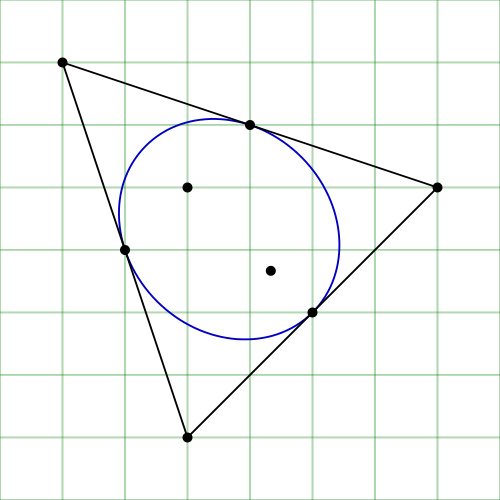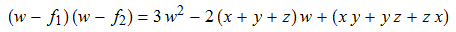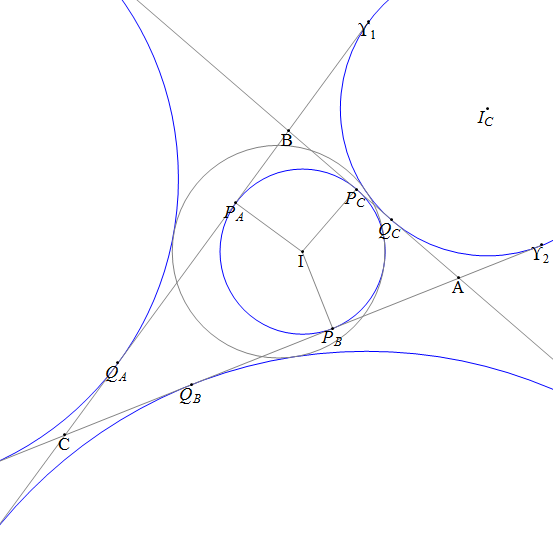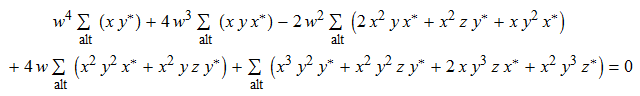# |cp4space| = 100

This is the 100th article on cp4space, and is inspired by a discussion last night with Gabriel Gendler.

### Triangle centres with complex numbers

Suppose we have a triangle in the Euclidean plane$\mathbb{R}^2$. We can identify the Euclidean plane with the complex plane, and represent the triangle by a set of three complex numbers (one for each vertex). I’ll call these complex numbers x, y and z (corresponding to vertices A, B and C, respectively). We’ll now consider a few interesting points in the plane of the triangle:The centroid, G, is the easiest triangle centre to express. It’s simply$G(x,y,z) = \frac{1}{3}(x+y+z)$, which is the arithmetic mean of the three vertices. Here, we consider triangle centres to be functions from$\mathbb{C}^3$ to$\mathbb{C}$, which takes the three vertices and returns that triangle centre.

The circumcentre, O, is somewhat more difficult to find. It’s the intersection of the three perpendicular bisectors (shown in red in the diagram above), and we can solve simultaneous linear equations to obtain an expression for the circumcentre.The orthocentre, H, and the nine-point centre, T, are expressible as simple linear combinations of O and G. For instance,$H(x,y,z) = 3G(x,y,z) - 2O(x,y,z)$ and$T(x,y,z) = \frac{1}{2}(H(x,y,z) + O(x,y,z))$. These points lie on the Euler line, as you can see in the diagram.

Finally, we can also get a simple expression for the symmedian point (either by considering areal coordinates or harmonic quadrilaterals, both of which are explained in MODA).

### Algebraically indistinguishable triangle centres

Consider the Steiner inellipse. This is the largest ellipse that can be contained within a triangle, or (equivalently) the image of the incircle of an equilateral triangle after it has been hit with an affine transformation to become the triangle of interest. Probably the easiest way to explain is with a diagram:Steiner inellipse — diagram courtesy of Claudio Rocchini

The diagram shows the two foci of the ellipse, f_1 and f_2. It is a remarkable fact that the quadratic polynomial with f_1 and f_2 as roots is the derivative of the cubic polynomial with x,y,z as roots. This gives us the following expression:Of course, we can now solve this quadratic:This is a multi-valued function, which gives both foci. It is impossible to create an algebraic expression that gives one focus but not the other; they are roots of the same minimal polynomial with coefficients in$\mathbb{Z}[x][y][z][x^{\star}][y^{\star}][z^{\star}]$. Points with this property (for general fields) are known as Galois conjugates.

The two foci in the previous expression are the same when the radicand (thing being square-rooted) is equal to zero, i.e. when$x^2 + y^2 + z^2 = xy + yz + zx$. This is equivalent to the triangle being equilateral.

### Tritangential circles

The foci of the Steiner inellipse constitute a pair of Galois conjugates. There are other interesting points that belong to larger sets of Galois conjugates. Consider the four circles that are tangent to all three sides of the triangle:The centres of these circles,$I, I_A, I_B, I_C$, are all roots of the same minimal polynomial, namely this horrible quartic in w:Here, I’ve used Σ_alt to indicate the alternating sum over permutations of (x, y, z). This is like the symmetric sum, but where the sign of each term is determined by the permutation of the parity. For example, the circumcentre is given by alt[x y y*]/alt[x y*].

### Transcendental triangle centres

The triangle centres (and other points) we’ve explored so far can be given as the roots of polynomials with coefficients in$\mathbb{Z}[x][y][z][x^{\star}][y^{\star}][z^{\star}]$. Some triangle centres, however, have no such expressions. One particularly interesting example is the Hofstadter point, X_360. Let the Cevian AX_360 intersect the line BC at D; this splits it in the ratio$BD : DC = \angle ABC : \angle BCA$. You can immediately spot that this is really bizarre, since a ratio between lengths is identified with a ratio between angles.

This entry was posted in Uncategorized. Bookmark the permalink.

### 0 Responses to |cp4space| = 100

1.wojowu says:

It’s 100th post! Yay! Lets talk about triangle centers!
Once I saw that you mentioned roots of quartics, I thought that equation for solving general quartic is something horrific enough to appear on CP4space. Is that equation being used anywhere? I think approximations have much more sense in that case

•apgoucher says:

I personally didn’t use Ferrari’s method to solve that quartic, since it’s better to leave it as a polynomial rather than express it in terms of a bunch of nested radicals.